Note: this document will print in an appropriately modified format (11 pages)

Although all gases closely follow the ideal gas law PV = nRT under appropriate conditions, each gas is also a unique chemical substance consisting of molecular units that have definite masses. In this lesson we will see how these molecular masses affect the properties of gases that conform to the ideal gas law.

Following this, we will look at gases that contain more than one kind of molecule— in other words, mixtures of gases.

We begin with a review of molar volume and the E.V.E.N. principle, which is central to our understanding of gas mixtures.

1  The molar volume of a gas

You will recall that the molar mass of a pure substance is the mass of 6.02 x 1023 (Avogadro's number) of particles or molecular units of that substance. Molar masses are commonly expressed in units of grams per mole (g mol–1) and are often referred to as molecular weights.

As was explained in the preceding lesson, equal volumes of gases, measured at the same temperature and pressure, contain equal numbers of molecules (this is the "EVEN" principle, more formally known as Avogadro's law.)

Standard temperature and pressure: 273K, 1 atm

This means that one mole of any gas will occupy the same volume as one mole of any other gas at a given temperature and pressure. The magnitude of this volume will of course depend on the temperature and pressure, so as a means of convenient comparison it is customary to define a set of conditions T = 273K and P = 1 atm as standard temperature and pressure, usually denoted as STP. Substituting these values into the ideal gas equation of state and solving for V yields a volume of 22.414 litres for 1 mole.

The standard molar volume 22.4 L mol –1 is a value worth memorizing, but remember that it is valid only at STP. The molar volume at other temperatures and pressures can easily be found by simple proportion.

The molar volume of a substance can tell us something about how much space each molecule occupies, as the following example shows.

#### Pure gases or mixtures: it makes no difference

Under conditions at which the ideal gas model is applicable (that is, almost always unless you are a chemical engineer dealing with high pressures), "a molecule is a molecule", so the volume of Avogadro's number of molecules will be independent of the composition of the gas. The reason, of course, is that the volume of the gas is mostly empty space; the volumes of the molecules themselves are negligible.

2  Molar mass and density of a gas

The molecular weight (molar mass) of any gas is the mass, expressed in grams, of Avogadro's number of its molecules. This is true regardless of whether the gas is composed of one molecular species or is a mixture.

For a mixture of gases, the molar mass will depend on the molar masses of its components, and on the fractional abundance of each kind of molecule in the mixture. The term "average molecular weight" is often used to describe the molar mass of a gas mixture.

### Average molecular weight of a gas

The average molar mass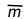of a mixture of gases is just the sum of the mole fractions xi of each gas, multiplied by the molar mass mi of that substance:

110-component gas mixture enters Guinness Book of Records in 2010. The U.S. branch of the Linde Corporation, which supplies specialty gases to industry, concocted this one to calibrate instruments that measure atmospheric pollution.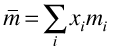### Density of a pure gas

The molar volumes of all gases are the same when measured at the same temperature and pressure. But the molar masses of different gases will vary. This means that different gases will have different densities (different masses per unit volume). If we know the molecular weight of a gas, we can calculate its density.

More importantly, if we can measure the density of an unknown gas, we have a convenient means of estimating its molecular weight. This is one of many important examples of how a macroscopic measurement (one made on bulk matter) can yield microscopic information (that is, about molecular-scale objects.)

Gas densities are now measured in industry by electro-mechanical devices such as vibrating reeds which can provide continuous, on-line records at specific locations, as within pipelines.

Determination of the molecular weight of a gas from its density is known as the Dumas method, after the French chemist Jean Dumas (1800-1840) who developed it. One simply measures the weight of a known volume of gas and converts this volume to its STP equivalent, using Boyle's and Charles' laws. The weight of the gas divided by its STP volume yields the density of the gas, and the density multiplied by 22.4 mol–1 gives the molecular weight. Pay careful attention to the examples of gas density calculations shown here and in your textbook. You will be expected to carry out calculations of this kind, converting between molecular weight and gas density.

3  Expressing the composition of a gas mixture

Because most of the volume occupied by a gas consists of empty space, there is nothing to prevent two or more kinds of gases from occupying the same volume. Homogeneous mixtures of this kind are generally known as solutions, but it is customary to refer to them simply as gaseous mixtures.

We can specify the composition of gaseous mixtures in many different ways, but the most common ones are by volumes and by mole fractions.

### Volume fractions in gas mixtures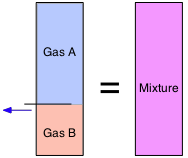From Avogadro's Law we know that "equal volumes contain equal numbers of molecules". This means that the volumes of gases, unlike those of solids and liquids, are strictly additive. So if a partitioned container has two volumes of gas A in one section and one volume of gas B in the other (both at the same temperature and pressure), and we remove the partition, the volume remains unchanged. We can specify the composition of this mixture by saying that the volume fraction of A is 2/3 and that of B is 1/3. Volume fractions are often called partial volumes:

Don't let this type of notation put you off! The summation sign Σ (Greek Sigma) simply means to add up the v's (volumes) of every gas. Thus if Gas A is the "i-th" substance as in the expression immediately above, the summation runs from i=1 through i=2.

Note that we can employ partial volumes to specify the composition of a mixture even if it had never actually been made by combining the pure gases.

When we say that air, for example, is 21 percent oxygen and 78 percent nitrogen by volume, this is the same as saying that these same percentages of the molecules in air consist of O2 and N2. Similarly, in 1.0 mole of air, there is 0.21 mol of O2 and 0.78 mol of N2 (the other 0.1 mole consists of various trace gases, but is mostly neon.)

Note that you could never assume a similar equivalence with mixtures of liquids or solids, to which the E.V.E.N. principle does not apply.

### Mole fractions in gas mixtures

These last two numbers (.21 and .78) also express the mole fractions of oxygen and nitrogen in air. Mole fraction means exactly what it says: the fraction of the molecules that consist of a specific substance. This is expressed algebraically by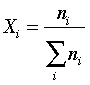so in the case of oxygen in the air, its mole fraction is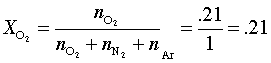4  Dalton's law of partial pressures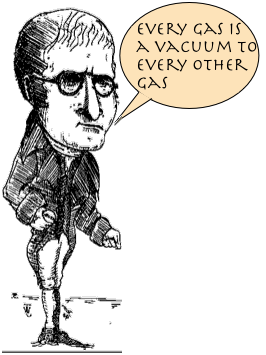The ideal gas equation of state applies to mixtures just as to pure gases. It was in fact with a gas mixture, ordinary air, that Boyle, Gay-Lussac and Charles did their early experiments. The only new concept we need in order to deal with gas mixtures is the partial pressure, a concept invented by the famous English chemist John Dalton (1766-1844). Dalton reasoned that the low density and high compressibility of gases indicates that they consist mostly of empty space; from this it follows that when two or more different gases occupy the same volume, they behave entirely independently.

The contribution that each component of a gaseous mixture makes to the total pressure of the gas is known as the partial pressure of that gas. Dalton himself stated this law in the simple and vivid way shown at the left.

The usual way of stating Dalton's Law of Partial Pressures is

The total pressure of a gas is the sum
of the partial pressures of its components

We can express this algebraically as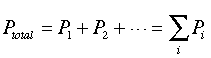or, equivalently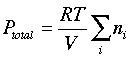(If you feel a need to memorize these formulas, you probably don't really understand Dalton's Law!)

There is also a similar relationship based on volume fractions, known as Amagat's law of partial volumes. It is exactly analogous to Dalton's law, in that it states that the total volume of a mixture is just the sum of the partial volumes of its components. But there are two important differences: Amagat's law holds only for ideal gases which must all be at the same temperature and pressure. Dalton's law has neither of these restrictions.

Although Amagat's law seems intuitively obvious, it sometimes proves useful in chemical engineering applications. We will make no use of it in this course.

5  Some applications of Dalton's law

### Collecting gases over water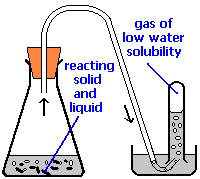A common laboratory method of collecting the gaseous product of a chemical reaction is to conduct it into an inverted tube or bottle filled with water, the opening of which is immersed in a larger container of water. This arrangement is called a pneumatic trough, and was widely used in the early days of chemistry. As the gas enters the bottle it displaces the water and becomes trapped in the upper part.

The volume of the gas can be observed by means of a calibrated scale on the bottle, but what about its pressure? The total pressure confining the gas is just that of the atmosphere transmitting its force through the water. (An exact calculation would also have to take into account the height of the water column in the inverted tube.) But liquid water itself is always in equilibrium with its vapor, so the space in the top of the tube is a mixture of two gases: the gas being collected, and gaseous H2O. The partial pressure of H2O is known as the vapor pressure of water and it depends on the temperature. In order to determine the quantity of gas we have collected, we must use Dalton's Law to find the partial pressure of that gas.

### Scuba diving and Dalton's law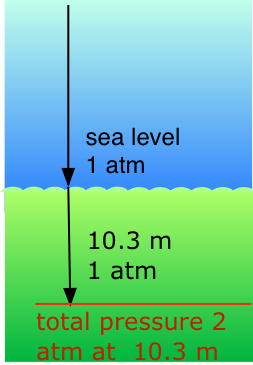Our respiratory systems are designed to maintain the proper oxygen concentration in the blood when the partial pressure of O2 is 0.21 atm, its normal sea-level value. Below the water surface, the pressure increases by 1 atm for each 10.3 m increase in depth; thus a scuba diver at 10.3 m experiences a total of 2 atm pressure pressing on the body. In order to prevent the lungs from collapsing, the air the diver breathes should also be at about the same pressure.

But at a total pressure of 2 atm, the partial pressure of O2 in ordinary air would be 0.42 atm; at a depth of 100 ft (about 30 m), the O2 pressure of .8 atm would be sufficiently high to induce oxygen toxicity. For this reason, the air mixture in the pressurized tanks that scuba divers wear must contain a smaller fraction of O2. This can be achieved most simply by raising the nitrogen content, but high partial pressures of N2 can also be dangerous, resulting in a condition known as nitrogen narcosis. The preferred diluting agent for sustained deep diving is helium, which has very little tendency to dissolve in the blood even at high pressures.

The Chemistry of Scuba Diving

Diving physics and "fizzyology"

Scuba diving and hyperbaric medicine

Concept Map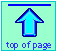Chem1 Moles, mixtures and densities of gases coversDalton's law of partial pressures and the E.V.E.N. prinipcle for a course in General Chemistry. It is part of the General Chemistry Virtual Textbook, a free, online reference textbook for General Chemistry by of of Simon Fraser University

This chapter covers the following topics:Molar vomue, molar mass and density of a gas, average molar mass of a mixture, gas density and its uses, volume fraction and mole fractions, Dalton's law and its applications to collecting gases over water, and scuba diving.. It can be accessed directly at .